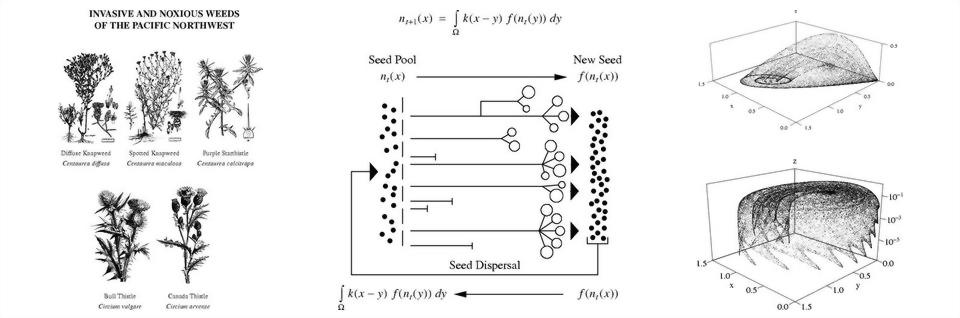# Research

My research is at the interface between applied mathematics and ecology and evolutionary biology. I model the dynamics of biological populations, use mathematics to shed light on the abundance and distribution of biological populations, and use biology to motivate interesting mathematical problems.

In recent years, my research has centered on integrodifference equations. These are discrete-time, continuous-space models for the growth and spread of biological populations. Integrodifference equations readily incorporate a range of dispersal mechanisms. They appear to be both more flexible and more realistic than simple reaction-diffusion models. Integrodifference equations are extremely useful for modeling biological invasions and for modelling the effects of climate change.

In addition to my long-standing interest in integrodifference equations, I am also interested in understanding how simple ecological models can give rise to complex or chaotic dynamics and in a broad range of other ecological and evolutionary models and analyses.

```
```